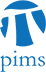## WENTS Seminar: On the correlation of shifted values of the Riemann zeta function

• Date: 03/04/2010
Lecturer(s):
Vorrapan Chandee, Stanford University
Location:

University of Calgary

Description:

In 2007, assuming the Riemann Hypothesis (RH), Soundararajan \cite{Moment}
proved that the 2k-th moments of the Riemann zeta function on the critical
line is bounded by T(log T)^{k^2 + epsilon} for every k positive real
number and every epsilon > 0.

In this talk I will generalize his methods to find upper bounds for
shifted moments. Also I will sketch the proof how we derive their lower
bounds and conjecture asymptotic formulas based on Random matrix model,
which is analogous to Keating and Snaith's work. These upper and lower
bounds suggest that the correlation of |\zeta(1/2 + it + i\alpha_1)| and
|\zeta(1/2 + it + i\alpha_2)| transition at |\alpha_1 - \alpha_2| is
around 1/log T}. In particular these distribution appear independent when
|\alpha_1 - \alpha_2| is much larger than 1/log T.

Schedule:

4:00 - 5:00pm (GMT-7), broadcast from ULeth to UC and UA.

Other Information:

This seminar series is an activity of the PIMS Collaborative Research Group in L-functions and Number Theory. See the official webpage at